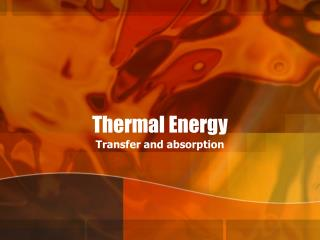DownloadDownload PresentationThermal Energy

# Thermal Energy

Télécharger la présentation## Thermal Energy

- - - - - - - - - - - - - - - - - - - - - - - - - - - E N D - - - - - - - - - - - - - - - - - - - - - - - - - - -
##### Presentation Transcript

1. Thermal Energy Transfer and absorption

2. Thermodynamics • Thermodynamics-study of heat transformations into other forms of energy

3. Heat • Fire dissipates heat • Does not absorb heat • Heat always flows from a hotter to cooler

4. Heat flowing from hotter to cooler

5. Grease fire • http://www.gocolumbiamo.com/Fire/Educational/stovefires.php • http://www.teachertube.com/view_video.php?viewkey=d76c5ec1acefe6d433b6 • http://www.teachertube.com/view_video.php?viewkey=e0da400d9b98c254b9fc

6. Thermal Energy • Thermal Energy- total energy of the molecules • Temperature- related to average energy per molecule

7. Thermal Energy and Temperature • Temperature-quantifies the average kinetic energy of the particles in an object • The warmer an object the more kinetic energy it has. • KEavg=KEt/(number of particles) • Thermal Energy- proportional to the number of particles

8. Equilibrium and Thermometry • Hot = particles move faster = more thermal energy • Conduction-transfer of kinetic energy when particles collide • energy transferred from a warmer object to a colder object • How much energy is in an object at 0 Kelvin (absolute zero)? • “Scientists put a light wave on hold” • http://www.msnbc.msn.com/id/3077366/

9. Equilibrium and Thermometry • Thermal Equilibrium- the state in which the rate of energy flow between two objects is equal and the objects are at the same temperature • Same temperature

10. Temperature Scales • Celsius- • The zero point is the freezing point of water • The 100 point is the boiling point of water • Kelvin • The zero point is where all thermal energy has been removed. No lower possible temperature, No motion in the atoms. Absolute Zero • 0 K =- 273.15 C • Tc + 273 = TK

11. Temperature Scales • Fahrenheit • The zero point is determined by a frigorific mixture of ice, water, and ammonium chloride (a salt). The mixture automatically stabilizes its temperature at 0 °F • The second point is the 32 degree found by putting the thermometer in still water as ice is just forming on the surface. • The third point, the 96 degree, was the level of the liquid in the thermometer when held in the mouth or under the armpit. (Has been since adjusted to 98.6)

12. Three Temperature Scales

13. Three Temperature Scales

14. Heat And how it transferred

15. Heat • Heat-energy that always flows from the hotter object to the cooler object. • “Left to itself heat never flows from a colder object to a hotter object.” • Variable ‘Q’ denotes heat • Q is in joules or J

16. Flow of Thermal Energy • Conduction • Convection • Radiation

17. Conduction • Heat is transferred (conducted) through particles that are in direct contact with each other.

18. Convection • The motion of fluid in a liquid or gas caused by temperature differences • Hotter fluids/gases rise. • Cooler fluids/gases sink. • “Real power from nothing but hot air” • http://www.guardian.co.uk/environment/2002/aug/19/energy.renewableenergy

19. Radiation • The transfer of energy by electromagnetic waves • Does not require contact • Travels at 3x10^8 m/s • Nuclear Test Site, Ground Zero

20. Specific Heat • The amount of energy that must be added to a material to raise the temperature of a unit mass by one temperature unit.

21. Heat Transfer • Q = mC∆T = mC(Tf –Ti) • Q = heat • m = mass • C = specific heat • T = temperature • Temperature intervals for Kelvin and Celsius are the same, (i.e. ∆T is the same) 3-5 , pg 319 Ex 1, pg318

22. Conservation of Energy • The total energy in an isolated, closed system never changes. • EA + EB = constant 6-7, pg321 Ex 2, pg321

23. Homework • Pg 322 • # 10, 12, 16, 17 • Pg 336 • #37, 39, 50, 52, 53, 57

24. Changes of State and the Laws of Thermodynamics • Change in state changes structure and the way in which atoms store thermal energy

25. States of matter • Solid • Liquid • Gas • Plasma

26. Change-of-State

27. Changes of State Heat = Q in Joules J • Melting Point • Heat of Fusion • Q=mHf • Boiling Point • Heat of Vaporization • Q=mHv • Condensation Point • Freezing Point

28. Thermal energy • Increase thermal energy • Increases particle speed • Causes more freedom of movement

29. Change-of-State All thermal energy added goes to overcome the forces holding the particles together. The temperature does not increase Boiling Melting

30. The First Law of Thermal Dynamics • Change in thermal energy is equal to the heat added minus the work done • ∆U=Q-W • Where • ∆U is the change in thermal energy • Q is the heat added • W is the work done

31. Efficiency • W/QH

33. The Second Law of Thermal Dynamics • Natural processes go in a direction that maintains of increases the total entropy of the universe. • Things get more disordered over time, assuming time is going forward.

34. The Animate and the Inanimate(available on class website) • Read Pg 4-8,42 • Explain what it means • One page write-up

35. Entropy • Entropy – the measure of disorder in a system • Change in Entropy – is equal to the heat added divided by the temperature in kelvins • ∆S=Q/T • The measure of unavailability of useful energy

36. Temperature and Thermal Energy • Describe thermal energy and compare it to potential and kinetic energies. • Distinguish between temperature and thermal energy